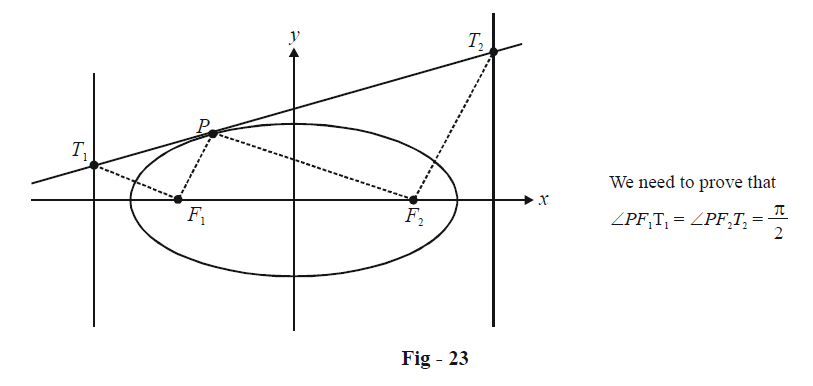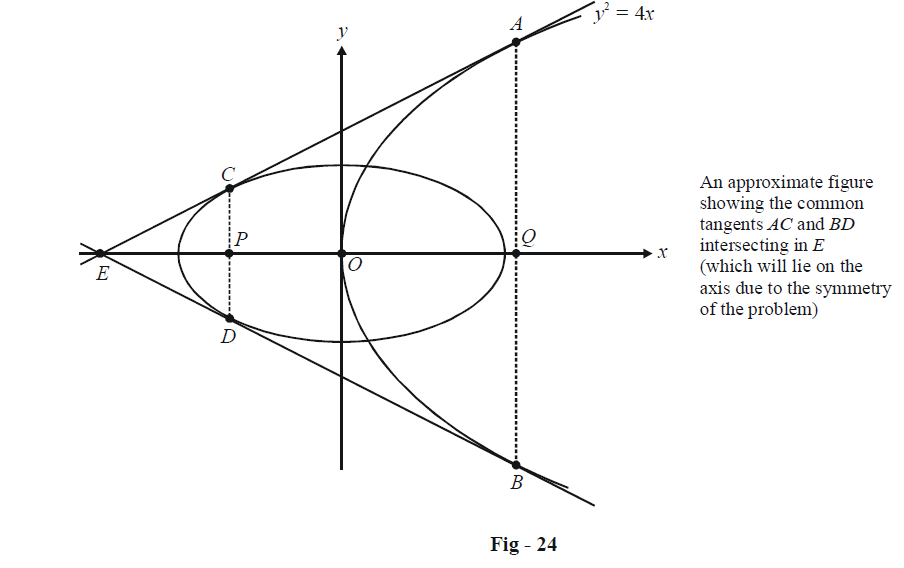# Examples On Tangents To Ellipses Set-3

Go back to  'Ellipse'

Example - 20

Prove that the portion of the tangent to any ellipse intercepted between the curve and a directrix subtends a right angle at the corresponding focus.

Solution: The following diagram makes the phrase “corresponding focus” clear:Let the equation of the ellipse be $$\frac{{{x^2}}}{{{a^2}}} + \frac{{{y^2}}}{{{b^2}}} = 1$$ and assume P to be $$(a\cos \theta ,\,b\sin \theta ).$$ F1 and F2 are (–ae, 0) and (ae, 0) respectively.

The equation of the tangent at P is

$\frac{x}{a}\cos \theta + \frac{y}{b}\sin \theta = 1$

T1 and T2 can now be evaluated since we know their x-coordinates as $$\frac{{ - a}}{e}and\frac{a}{e}$$ respectively.

\begin{align}&{T_1}: \quad x = \frac{{ - a}}{e} & & \Rightarrow & y = \frac{{b(e + \cos \theta )}}{{e\sin \theta }}\\& \Rightarrow \quad {T_1} \equiv \left( {\frac{{ - a}}{e},\,\frac{{b(e + \cos \theta )}}{{e\sin \theta }}} \right)\end{align}

Similarly,

${T_2} \equiv \left( {\frac{a}{e},\,\frac{{b(e - \cos \theta )}}{{e\sin \theta }}} \right)$

Now we evaluate the appropriate slopes:

\begin{align}&{m_{{F_1}{T_1}}} = \frac{{\frac{{b(e + \cos \theta )}}{{e\sin \theta }}}}{{ae - \frac{a}{e}}} = \frac{{ - b(e + \cos \theta )}}{{a\sin \theta (1 - {e^2})}}\\&{m_{P{F_1}}} = \frac{{b\sin \theta }}{{a\cos \theta + ae}} = \frac{{b\sin \theta }}{{a(e + \cos \theta )}}\\ &\Rightarrow\quad {m_{{F_1}{T_1}}} \times {m_{P{F_1}}} = \frac{{ - {b^2}}}{{{a^2}(1 - {e^2})}} = \frac{{ - {b^2}}}{{{b^2}}} = - 1\end{align}

which implies $${F_1}{T_1} \bot P{F_1}$$

Similarly, we can show that $${F_2}{T_2} \bot P{F_2}.$$

Thus, the two intercepts subtend right angles at their corresponding foci.

Example - 21

Common tangents are drawn to the parabola $${y^2} = 4x$$ and the ellipse $$\frac{{{x^2}}}{{16}} + \frac{{{y^2}}}{6} = 1$$ touching the parabola at A and B and the ellipse at C and D. What is the area of the quadrilateral ABDC ?

Solution:Any tangent to the parabola $${y^2} = 4x$$ can be written in the form

$y = mx + \frac{1}{m}$

This line touches the ellipse if the condition for tangency, $${c^2} = {a^2}{m^2} + {b^2}$$ is satisfied, i.e. if

$\frac{1}{{{m^2}}} = 10{m^2} + 6$

giving $m = \pm \frac{1}{{2\sqrt 2 }}$

Thus, the two tangents AE and BE are

$y = \pm \left( {\frac{1}{{2\sqrt 2 }}x + 2\sqrt 2 } \right)$

which evidently intersect at $$E( - 4,\,0).$$

The point of contact for the parabola $${y^2} = 4ax$$ is given by $$\left( {\frac{a}{{{m^2}}},\,\frac{{2a}}{m}} \right).$$ Thus A and B have the coordinates $$(8,\, \pm 4\sqrt 2 )$$ so that

$AB = 8\sqrt 2$

The point of contact for the ellipse will be $$\left( { \mp \frac{{{a^2}m}}{{\sqrt {{a^2}{m^2} + {b^2}} }},\,\, \pm \frac{{{b^2}}}{{\sqrt {{a^2}{m^2} + {b^2}} }}} \right).$$

Thus, C and D will have the coordinates $$\left( { - 2,\,\, \pm \frac{3}{{\sqrt 2 }}} \right)$$  so that

$CD = 3\sqrt 2$

Finally, PQ can now easily be seen to be 8 + 2 = 10. The area of quadrilateral ABDC (which is actually a trapezium) is

\begin{align}&\Delta = \frac{1}{2} \times (AB + CD) \times PQ\\&\quad{\rm{ }} = 55\sqrt 2 {\rm{ sq units}}\end{align}